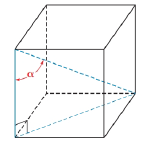Chapter 11.2, Problem 37E### Elementary Geometry for College St...

6th Edition
Daniel C. Alexander + 1 other
ISBN: 9781285195698

#### Solutions

Chapter
Section### Elementary Geometry for College St...

6th Edition
Daniel C. Alexander + 1 other
ISBN: 9781285195698
Textbook Problem
1 views

# In Exercise 29 to 37, angle measures should be given to the nearest degree; distances should be given to the nearest tenth of a unit.What is the size of the angle α formed by a diagonal of a cube and one of its edges?.To determine

To find:

To find size of the angle α formed by a diagonal of a cube and one of its edges.

Explanation

Consider the following figure,

General formula for cosine ratio and sin ratio is given below,

Let the side length of the cube be x units.

Then the diagonal formed by the base of the cube is 2x units

### Still sussing out bartleby?

Check out a sample textbook solution.

See a sample solution

#### The Solution to Your Study Problems

Bartleby provides explanations to thousands of textbook problems written by our experts, many with advanced degrees!

Get Started

#### Find more solutions based on key concepts